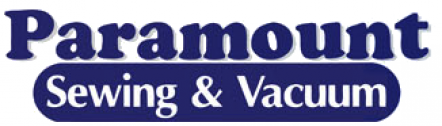## Lessons - Eugene

Basic machine instruction

Cost: \$ FREE
Basic machine instruction

Cost: \$ FREE
Basic machine instruction

Cost: \$ FREE
Basic machine instruction

Cost: \$ FREE
Basic machine instruction

Cost: \$ FREE
Basic machine instruction

Cost: \$ FREE
Basic machine instruction

Cost: \$ FREE
Basic machine instruction

Cost: \$ FREE
Basic machine instruction

Cost: \$ FREE
Basic machine instruction

Cost: \$ FREE
Basic embroidery machine instruction

Cost: \$ FREE

Basic embroidery machine instruction

Cost: \$ FREE
Basic embroidery machine instruction

Cost: \$ FREE

Basic embroidery machine instruction

Cost: \$ FREE

Basic embroidery machine instruction

Cost: \$ FREE
Basic embroidery machine instruction

Cost: \$ FREE
Basic machine instruction

Cost: \$ FREE
Basic embroidery machine instruction

Cost: \$ FREE

Basic machine instruction

Cost: \$ FREE
Basic embroidery machine instruction

Cost: \$ FREE

Basic machine instruction

Cost: \$ FREE
Basic embroidery machine instruction

Cost: \$ FREE

Basic machine instruction

Cost: \$ FREE
Basic embroidery machine instruction

Cost: \$ FREE

Basic machine instruction

Cost: \$ FREE
Basic embroidery machine instruction

Cost: \$ FREE

Basic machine instruction

Cost: \$ FREE
Basic embroidery machine instruction

Cost: \$ FREE

Basic machine instruction

Cost: \$ FREE
Basic embroidery machine instruction

Cost: \$ FREE
Basic machine instruction

Cost: \$ FREE
Basic machine instruction

Cost: \$ FREE
Basic embroidery machine instruction

Cost: \$ FREE